#### Number of problems found: 2612

• Amazing numberAn amazing number is name for such even number, the decomposition product of prime numbers has exactly three not necessarily different factors and the sum of all its divisors is equal to twice that number. Find all amazing numbers.
• RingThe ring made from gold and copper alloy has a weight of 14.5 g and a volume of 1.03 cm3. How much gold and how much copper it contains? The metal densities are Au 19.3 g/cm³ and Cu 8.94 kg·dm-3
• The rectangleDetermine the area of the rectangle where the length and width are in the ratio 5:2 and its length is 7.5 cm longer than its width. Determine also its length and its width.
• Fog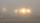The car started in fog at speed 30 km/h. After a 12-minute drive, the fog dissipated and the driver drove next 12 minutes distance 17 km. On the last 17 km long again the driving conditions deteriorated and the driver drove the speed of 51 km/h. a) CalculMarie wants to make a cone-shaped witch's hat for a masquerade ball. How much material will it need if it counts on an annular rim with diameters of 28cm and 44cm? Hat side length is 30cm. Add 5% of the material to the bust. Round to cm2.
• Diagonal 20Diagonal pathway for the rectangular town plaza whose length is 20 m longer than the width. if the pathway is 20 m shorter than twice the width. How long should the pathway be?
• CylinderIn a 1-meter diameter cylinder is 1413 liters of water, which is 60% of the cylinder. Calculate the cylinder height in meters, do not write the units. The resulting value round and write as an integer.
• Candelas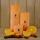We burned two unequally thick and long candles. Longer burnt for three and a half hours and shorter for five hours. After two hours of burning it was identical. How many times was longer candle longer then shorter?
• Three granaries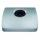Three granaries hold 7,800 metric cent (q) of grain. In the first, 70 tonnes of grain was more than the second and in the third it was 120 tonnes less than in the second. How many grain were in each granary?
• Passenger car and truckFrom Kutna Hora left at 11:00 clock a truck at 60 km/h. At 12:30 behind him started passenger car at average speed 80 km/h. At what time and how far from Kutna Hora overtake a passenger car truck?
• Aluminum wireAluminum wire of 3 mm diameter has a total weight of 1909 kg and a density of 2700 kg/m3. How long is the wire bundle?
• Water reservoir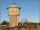The reservoir shape of a cylinder having a diameter 8 m and a height 2.8 m. The device indicates that the reservoir contains 800 hectoliters of water. Calculate how much % of volume is used.
• TreeBetween points A and B is 50m. From A we see a tree at an angle 18°. From point B we see the tree in three times bigger angle. How tall is a tree?
• Roses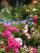On the large rosary was a third white, half red, yellow quarter and six pink. How many roses was in the rosary?
• CostumeDenisa is preparing for a goldsmith's costume carnival. During the preparations, she thought she would let her hair wipe instead - she would apply a 5 μm thick layer of gold to each hair. How much gold would Denisa need? Assume that all hundred thousand D
• Trees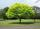Loggers wanted to seed more than 700 and less than 800 trees. If they seed in rows of 37, left them 8 trees. If they seed in rows of 43, left the 11 trees. How many trees must seed ?
• Equilateral triangle v2Equilateral triangle has a perimeter 36 dm. What is its area?
• Train speedTwo guns were fired from the same place at an interval of 10 minutes and 30 seconds, but a person in a train approaching the place hears second shot 10 minutes after the first. The speed of the train (in km/hr), supposing that sound travels at 340 m/s is:
• Journey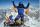The road from A to B measures 11.5 km. Firstly up the hill, then by level plane and then downhill. Tourist goes uphill at 3 km/h, on the plane 4 km/h and downhill 5 km/h. From point A to B went 2h 54 min back 3h 6 min. How long is the segment of level pla
• Equilateral triangle ABCIn the equilateral triangle ABC, K is the center of the AB side, the L point lies on one-third of the BC side near the point C, and the point M lies in the one-third of the side of the AC side closer to the point A. Find what part of the ABC triangle cont

Do you have an interesting mathematical word problem that you can't solve it? Submit a math problem, and we can try to solve it.

We will send a solution to your e-mail address. Solved examples are also published here. Please enter the e-mail correctly and check whether you don't have a full mailbox.

Please do not submit problems from current active competitions such as Mathematical Olympiad, correspondence seminars etc...